## Boolean-Algebra

 Question 1

Consider the Boolean operator with the following properties:Then x#y is equivalent to

 AB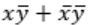C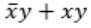DDigital-Logic-Design       Boolean-Algebra       GATE 2016 [Set-1]       Video-Explanation
Question 1 Explanation: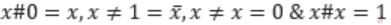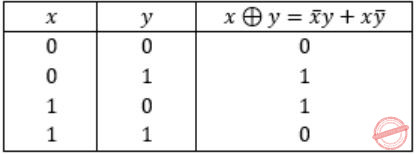Ex-OR satisfies all the properties. Hence,Question 2

Let, x1⊕x2⊕x3⊕x4 = 0 where x1, x2, x3, x4 are Boolean variables, and ⊕ is the XOR operator. Which one of the following must always be TRUE?

 A x1x2x3x4 = 0 B x1x3+x2 = 0 CD x1 + x2 + x3 + x4 = 0
Digital-Logic-Design       Boolean-Algebra       GATE 2016 [Set-2]       Video-Explanation
Question 2 Explanation:
Given expression is,
x1 ⊕ x2 ⊕ x3 ⊕ x4 = 0 -----(1)
A) x1x2x3 x4 = 0
Put x1 = 1, x2 = 1, x3 = 1, x4 = 1
The given equation will be zero, i.e.,
1 ⊕ 1 ⊕ 1 ⊕ 1 = 0
But,
x1x2x3 x4 ≠ 0
So, false.
B) x1x3 + x2 = 0
Put x1 = 1, x2 = 1, x3 = 0 , x4 = 0
The given equation will be zero, i.e.,
1 ⊕ 1 ⊕ 0 ⊕ 0 = 0
But,
x1x3 + x2 ≠ 0
So, false.
D) x1 + x2 + x3 + x4 = 0
Let x1=1, x2=1, x3=0, x4=0
The given equation will be zero, i.e.,
1 ⊕ 1 ⊕ 0 ⊕ 0 = 0
But,
x1 + x2 + x3 + x4 ≠ 0
So, false.
(i) True.
 Question 3

The number of min-terms after minimizing the following Boolean expression is ______.

`      [D′ + AB′ + A′C + AC′D + A′C′D]′    `
 A 1 B 2 C 3 D 4
Digital-Logic-Design       Boolean-Algebra       GATE 2015 [Set-2]
Question 3 Explanation:
Lets simplify it
[D' + AB' + A'C + AC'D + A'C'D]'
[D' + AB' + A'C + C'D (A + A')']' (since A+A' = 1)
[AB' + A'C + (D' + C') (D' + D)]' (since D' + D =1)
[AB' + A'C + D' + C']'
[AB' + (A' + C') (C + C') + D']'
[AB' + A' + C' + D']'
[(A + A') (A' + B') + C' + D']'
[A' + B' + C' + D']'
Apply de-morgan's law,
ABCD
 Question 4

Consider the following Boolean expression for F:

`   F(P, Q, R, S) = PQ + P'QR + P'QR'S`

The minimal sum-of-products form of F is

 ABC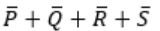DDigital-Logic-Design       Boolean-Algebra       GATE 2014 [Set-1]
Question 4 Explanation:
PQ + P’QR + P’QR’S
= Q(P+P’R) + P’QR’S
= Q(P+R) + P’QR’S
= QP + QR + P’QR’S
= QP + Q(R + P’R’S)
= QP + Q( R + P’S)
= QP + QR + QP’S
= Q(P+P’S) + QR
= Q(P+S)+ QR
= QP + QS + QR
 Question 5

The truth tablerepresents the Boolean function

 A X B X + Y C X ⊕ Y D Y
Digital-Logic-Design       Boolean-Algebra       GATE 2012
Question 5 Explanation:
f(X,Y) = XY’ + XY = X(Y’ + Y) = X
 Question 6

The simplified SOP (sum of product) form of the boolean expressionABCDDigital-Logic-Design       Boolean-Algebra       GATE 2011
Question 6 Explanation:Question 7

The minterm expansion of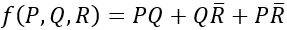is

 A m2+m4+m6+m7 B m0+m1+m3+m5 C m0+m1+m6+m7 D m2+m3+m4+m5
Digital-Logic-Design       Boolean-Algebra       GATE 2010
Question 7 Explanation:
Convert PQ + QR' + PR' into canonical form
= PQR + PQR' + PQR' + P'QR' + PQR' + PQ'R'
= PQR + PQR' + P'QR' + PQ'R'
= m7 + m6 + m2 + m4
 Question 8

If P, Q, R are Boolean variables, thenSimplifies to

 ABC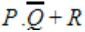D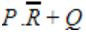Digital-Logic-Design       Boolean-Algebra       GATE 2008
Question 8 Explanation:Question 9

What is the maximum number of different Boolean functions involving n Boolean variables?

 A n2 B 2n C 22n D 2n2
Digital-Logic-Design       Boolean-Algebra       GATE 2007
Question 9 Explanation:
Each “boolean” variable has two possible values i.e 0 and 1.
Number of variables= n
Number of input combinations is 2n.
Each “boolean” function has two possible outputs i.e 0 and 1.
Number of boolean functions possible is 22n.
Formula: The number of m-ary functions possible with n k-ary variables is mkn.
 Question 10

The Boolean function x'y' + xy + x'y is equivalent to

 A x' + y' B x + y C x + y' D x' + y
Digital-Logic-Design       Boolean-Algebra       GATE 2004
Question 10 Explanation:
x'y' + xy + x'y
= x'y' + x'y + xy
= x'(y'+y)+xy
= x'⋅1+xy
= x'+xy
= (x'+x)(x'+y)
= 1⋅(x'+y)
= x'+y
 Question 11

The simultaneous equations on the Boolean variables x, y, z and w,

```      x + y + z = 1
xy = 0
xz + w = 1
xy +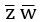= 0```

have the following solution for x, y, z and w, respectively.

 A 0 1 0 0 B 1 1 0 1 C 1 0 1 1 D 1 0 0 0
Digital-Logic-Design       Boolean-Algebra       GATE 2000
Question 11 Explanation:
Just put the values of each options in the equation and check it.
 Question 12

What values of A, B, C and D satisfy the following simultaneous Boolean equations?A A = 1, B = 0, C = 0, D = 1 B A = 1, B = 1, C = 0, D = 0 C A = 1, B = 0, C = 1, D = 1 D A = 1, B = 0, C = 0, D = 0
Digital-Logic-Design       Boolean-Algebra       GATE 1995
Question 12 Explanation:
For verification, just put up the values and check for AND, OR operations and their outputs.
 Question 13
Any set of Boolean operators that is sufficient to represent all Boolean expressions is said to be complete. Which of the following is not complete?
 A { AND, OR } B { AND, NOT } C { NOT, OR } D { NOR }
Digital-Logic-Design       Boolean-Algebra       ISRO-2018       Video-Explanation
Question 13 Explanation:
→ NOT, AND and OR gates are basic gates
→ NAND and NOR gates are universal gates.
→ With the help of universal gates, we can construct any boolean expressions. These gates are also called functionally complete.
→ AND+NOT=NAND→ Functionally Complete
→ OR+NOT=NOR→ Functionally Complete
→ NOR→ Functionally Complete
→ AND+OR→ Not functionally complete
 Question 14
The Boolean theorem AB + A’C + BC = AB + A’C corresponds to
 A (A + B) ∙ (A’ + C) ∙ (B + C) = (A + B) ∙ (A’ + C) B AB + A’C + BC = AB + BC C AB + A’C + BC = (A + B) ∙ ( A ‘+ C) ∙ (B + C) D (A + B) ∙ (A’ + C) ∙ (B + C) = AB + A’C
Digital-Logic-Design       Boolean-algebra       ISRO CS 2008
Question 14 Explanation:
(X+Y)*(X+Z)*(Y+Z)=(X+Y)*(X+Z) Consensus Law
XY+XZ+YZ=XY+XZ Consensus Law

For min-term: AB + A'C +BC = AB + A'C
AND for max-term : ( A + B ).( A' + C ).( B + C ) = ( A + B ).(A' + C )
 Question 15
In the given network of AND and OR gates, f can be written as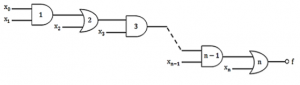A X0X1X2 … Xn + X1X2 … Xn + X2X3 … Xn + ⋯ + Xn B X0X1 + X2X3 + … Xn-1 Xn C X0 + X1 + X2 + … + Xn D X0X1 + X3 … Xn−1 + X2X3 + X5 … Xn−1 + ⋯ + Xn−2Xn−1 + Xn E None of the above
Digital-Logic-Design       Boolean-algebra       ISRO CS 2008
Question 15 Explanation:
(X0X1+X2)X3+X4)X5+⋯+XN
=(X0X1X3+X2X3+X4)X5+⋯+XN

=X0X1X3X5+X2X3X5+X4X5+⋯+XN

=X0X1X3X5⋯XN−1+X2X3X5⋯XN−1+X4X5X7⋯XN−1+⋯+XN
 Question 16
The Boolean expression ( A + C’)(B’+ C’) simplifies to
 A C’ + AB’ B C’ (A’ + B) C B’C’ + AB’ D None of these.
Digital-Logic-Design       Boolean-algebra       ISRO CS 2008
Question 16 Explanation:

The following expression can be simplified as:

(A + C')(B'+ C')

= AB' + AC' + B'C' + C
'
= AB' + C'(A + B' + 1) // 1 + A = 1

= AB' + C'
 Question 17
In the expression A'(A’ + B’) by writing the first term A as A + 0, the expression is best simplified as
 A A + AB B AB C A' D A + B
Digital-Logic-Design       Boolean-Algebra       ISRO CS 2008
Question 17 Explanation:

A'(A’ + B’)

This expression can be simplified as:

= A'A' + A'B'

= A' + A'B'

= A'(1 + B') // 1 + B' = 1

= A'
 Question 18
Which of the following is not a valid rule of XOR?
 A 0 XOR 0 = 0 B 1 XOR 1 = 1 C 1 XOR 0 = 1 D B XOR B = 0
Digital-Logic-Design       Boolean-Algebra       ISRO CS 2008
Question 18 Explanation:

XOR gate only returns 1 as the output when both inputs are different and in every other case, it returns 0.

So, options (A), (C) and (D) are correct.
 Question 19
In Boolean algebra, rule (X+Y)(X+Z) =
 A Y+XZ B X+YZ C XY+Z D XZ+Y
Digital-Logic-Design       Boolean-Algebra       ISRO CS 2011
Question 19 Explanation:
in Boolean algebra,
(X+Y)(X+Z) = X + XZ + XY + YZ
= X(1 + Z + Y) + YZ // as (1 + A = A)
= X.1 + YZ
= X + YZ
 Question 20
Which logic gate is used to detect overflow in 2’s complement arithmetic?
 A OR gate B AND gate C NAND gate D XOR gate
Digital-Logic-Design       Boolean-algebra       ISRO CS 2013
Question 20 Explanation: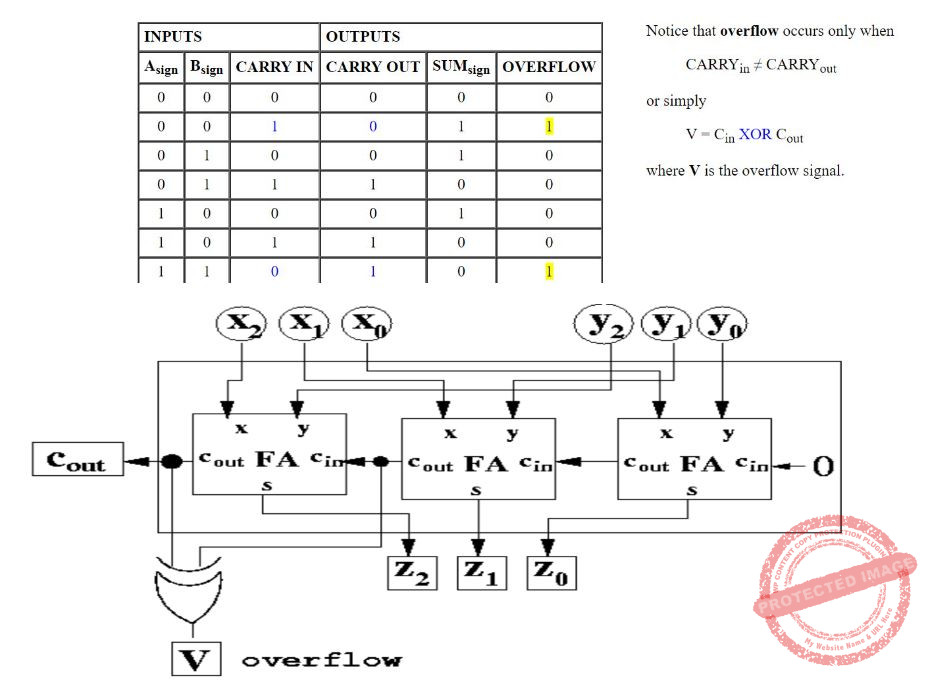Question 21
Which of the following is not valid Boolean algebra rule?
 A X.X = X B (X + Y).X = X C X̄ + XY = Y D (X + Y).(X + Z) = X + YZ
Digital-Logic-Design       Boolean-Algebra       ISRO CS 2014
Question 21 Explanation:
i) X.X = X
ii) (X + Y).X
= X.X + X.Y
= X + X.Y
= X(1 + Y)
= X
iii) X' + XY
= (X' + X)(X' + Y)
= (1)(X' + Y)
= (X' + Y)
iv) (X + Y).(X + Z)
= X.X + X.Z + X.Y + Y.Z
= X(1 + Z + Y) + Y.Z
= X + Y.Z
 Question 22

Perform the following operation for the binary equivalent of the decimal numbers

(-14)10 + (-15)10

The solution in 8 bit representation is :

 A 11100011 B 00011101 C 10011101 D 11110011
Digital-Logic-Design       Boolean-Algebra       UGC-NET CS 2018 JUNE Paper-2
Question 22 Explanation:
(-14)10 + (-15)10 = (-29)10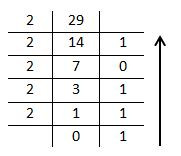(29)10 = (11101)2
(29)10 in 8-bit representation = (00011101)2(-29)10 = (00011101)2
 Question 23
In digital logic, if A B=C, then which one of the following is true?
 A A ⊕ C=B B B ⊕ C=A C A ⊕ B ⊕ C=0 D Both A) and B)
Digital-Logic-Design       Boolean-Algebra       Nielit Scientist-B CS 22-07-2017
Question 23 Explanation:Question 24
Which of the following logic expression is incorrect?
 A 1 ⊕ 0=1 B 1 ⊕ 1 ⊕ 0=1 C 1 ⊕ 1 ⊕ 1=1 D 1 ⊕ 1 =0
Digital-Logic-Design       Boolean-Algebra       Nielit Scientist-B CS 2016 march
Question 24 Explanation:
This ​ ⊕ ​ symbol is nothing but Ex-OR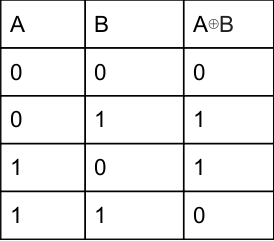Option A: Here, ​ 1 ⊕ 0=1 true according to truth table.
Option B: 1⊕1=0⊕0=0 false
Option C: 1 ⊕ 1=0 ⊕ 1=1 true
Option D: 1 ⊕ 1 =0 true.
 Question 25

The boolean expression A'⋅B + A⋅B' + A⋅B is equivalent to

 A A+B B A⋅B C (A+B)' D A'⋅B
Digital-Logic-Design       Boolean-Algebra       UGC-NET CS 2018 DEC Paper-2
Question 25 Explanation:Question 26

The relation ≤ and < on a boolean algebra are defined as :

```x ≤ y and only if x ∨ y = y
x < y means x ≤ y but x ≠ y
x ≥ y means y ≤ x and
x > y means y < x```

Consider the above definitions, which of the following is not true in the boolean algebra ?

(i) If x ≤ y and y ≤ z, then x ≤ z
(ii) If x ≤ y and y ≤ x, then x = y
(iii) If x < y and y < z, then x ≤ y
(iv) If x < y and y < z, then x < y

Choose the correct answer from the code given below:

Code:
 A (iv) only B (iii) only C (i) and (ii) only D (ii) and (iii) only
Digital-Logic-Design       Boolean-Algebra       UGC-NET CS 2018 DEC Paper-2
Question 26 Explanation:
iii) “If x < y and y < z, then x ≤ y” is not true.
Because x < y means x ≤ y but x ≠ y.
ii) If x ≤ y and y ≤ x, then x = y is true
Because
x ≤ y implies x v y = x
y ≤ x implies x v y = y
x v y = x = y
 Question 27

Consider the following boolean equations :

```(i) wx + w(x + y) + x(x + y)= x + wy
(ii) (wx’(y + xz’) + w’x’)y = x’y```

What can you say about the above equations ?

 A Both (i) and (ii) are true B (i) is true and (ii) is false C Both (i) and (ii) are false D (i) is false and (ii) is true
Digital-Logic-Design       Boolean-Algebra       UGC-NET CS 2018 DEC Paper-2
Question 27 Explanation:
(i)
wx + w(x + y) + x(x + y)
= (wx + wx) + wy + (x + xy)
= wx + wy + x(1 + y)
= wx + wy + x
= (w + 1)x + wy
= x + wy
(ii)Question 28

Find the boolean expression for the logic circuit shown below :

(1-NAND gate, 2-NOR gate, 3-NOR gate)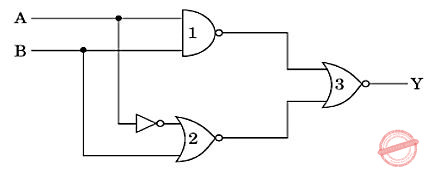A AB B AB’ C A’B’ D A’B
Digital-Logic-Design       Boolean-Algebra       UGC-NET CS 2018 DEC Paper-2
Question 28 Explanation: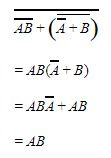Question 29

Which of the following statements are true ?

(i) Every logic network is equivalent to one using just NAND gates or just NOR gates.
(ii) Boolean expressions and logic networks correspond to labelled acyclic digraphs.
(iii) No two Boolean algebras with n atoms are isomorphic.
(iv) Non-zero elements of finite Boolean algebras are not uniquely expressible as joins of atoms.

Choose the correct answers from the code given below :

Code :
 A (i) and (iv) only B (i) and (ii) only C (i), (ii) and (iii) only D (ii), (iii) and (iv) only
Digital-Logic-Design       Boolean-Algebra       UGC-NET CS 2018 DEC Paper-2
Question 29 Explanation:
→ A universal logic gate is a logic gate that can be used to construct all other logic gates.
→ NAND and NOR are universal gates, by using these gates we can construct all gates.
→ An atom of a Boolean algebra is an element x such that there exist exactly two elements y satisfying y ≤ x, namely x and 0. A Boolean algebra is said to be atomic when every element is a sup of some set of atoms (the bottom element is always the empty sup).
→ So, the options (iii) and (iv) are false.
 Question 30
​ In digital logic, if A ​ ⊕ ​ B=C, then which one of the following is true?
 A A ​ ⊕ ​ C=B B B ​ ⊕ ​ C=A C A ​ ⊕ ​ B ​ ⊕ ​ C=0 D Both A) and B) E None of these
Digital-Logic-Design       Boolean-Algebra       Nielit Scientist-B IT 22-07-2017
Question 30 Explanation:
Given that
XOR:
X ⊕ X= 0
X ⊕ X'= 1
X ⊕ 0 = X
X ⊕ 1 = X'
A) A ⊕ C = B
A ⊕ A ⊕ B = B
0 ⊕ B = B
B= B
B) B ⊕C = A
B ⊕ A ⊕ B = A
A ⊕ 0=A
A=A
C) A⊕B=C
A⊕B⊕ C=C ⊕C ,
A⊕B⊕ C= 0
All are correct
 Question 31

Which of the following does NOT represent the Exclusive NOR operation over the binary variables A and B?

 A A’ ⊕ B’ B A ⊕ B’ C A’ ⊕ B D AB + A’B’
Digital-Logic-Design       Boolean-Algebra       JT(IT) 2018 PART-B Computer Science
Question 31 Explanation:
A’ ⊕ B’ = Ex-NORQuestion 32
The term sum of product in boolean algebra means
 A The AND function of several AND functions B The AND function of several OR functions C The OR function of several AND functions D The OR function of several OR functions
Digital-Logic-Design       Boolean-Algebra       KVS DEC-2017
Question 32 Explanation:
Sum of product: A boolean expression consisting purely of Minterms (product terms) is said to be in canonical sum of products form.
Example: F=AB+AC+AD Product of sum: A boolean expression consisting purely of Maxterms (sum terms) is said to be in canonical product of sums form.
Example: F=(A+B).(A+C).(A+D)
 Question 33

In boolean algebra, (x ⋀ y)’ = x’ V y’ and (x V y)’ = x’ ⋀ y’ is known as ___ law.

 A Demorgan’s law B Absorption C Dominance D Idempotent
Digital-Logic-Design       Boolean-Algebra       JT(IT) 2016 PART-B Computer Science
Question 33 Explanation:
The rules can be expressed in English as:
1. The negation of a disjunction is the conjunction of the negations.
2. The negation of a conjunction is the disjunction of the negations.
 Question 34
The boolean expression (A+C)(AB'+AC)(AC'+B') can be simplified as
 A A'B+BC B AB' C AB+BC D AB+A'C
Digital-Logic-Design       Boolean-Algebra       KVS DEC-2017
Question 34 Explanation:
(A+C)(AB'+AC)(AC'+B')
First compute one and primes
(AAB'+AAC+AB'C+ACC)(AC'+B')
to take common AC then we get
(AB'+AC+AB'C+AC)(AC'+B')
To take common AB'
(AB'(1+C)+AC)(AC'+B')
(AB'+AC)(AC'+B')
AB'AC'+AB'B'+ACAC'+ACB'
AB'C'+AB'+0+ACB'
AB'(C'+1)+ACB'
AB'+ACB'
AB'(1+C)
AB'
(or)
We can also use truth table to get same solution.
 Question 35
How many gates would be required to implement the following boolean expression after simplification? Expression:A 4 B 6 C 8 D 10
Digital-Logic-Design       Boolean-Algebra       KVS 30-12-2018 Part B
Question 35 Explanation:
Given expression is =A’+B’+C’+A’C+B’C+A’BC’+AB’+AC’+AB’C
=A’+A’C+B’+B’C+C’+AC’+A’BC’+AB’+AB’C
=A’ (1+C)+B’(1+C)+C’(1+A)+A’BC’+AB’(1+C) [ 1+X=1]
=A’+B’+C’+A’BC’+AB’
=A’+C’+A’BC’+B’ (1+A)
=A’+C’+A’BC’+B’
=A’+B’+C’ (1+A’B)
=A’+B’+C’
Total four gates required three not gates and one OR gate.
 Question 36
A boolean operator θ is defined as follows:
1θ1=1
1θ0=0
0θ1=0
0θ0=1
What will be the truth value of the expression (XθY)θZ=Xθ(YθZ)?
 A Always false B Always true C Sometimes true D Sometimes false
Digital-Logic-Design       Boolean-Algebra       KVS 30-12-2018 Part B
Question 36 Explanation:
(XθY)θZ=Xθ(YθZ) is always true if θ is associative.
From the given table, we can infer that θ is Ex-NOR operator.
Ex-NOR is associative. Hence (XθY)θZ=Xθ(YθZ) is always true
 Question 37
Consider the graph given below :Use Kruskal’s algorithm to find a minimal spanning tree for the graph. The List of the edges of the tree in the order in which they are chosen is?
 A AD, AE, AG, GC, GB, BF B GC, GB, BF, GA, AD, AE C GC, AD, GB, GA, BF, AE D AD, AG, GC, AE, GB, BF
Digital-Logic-Design       Boolean-Algebra       UGC NET CS 2017 Nov- paper-2
Question 37 Explanation:
Kruskal’s algorithm will sort all edge weight first and according to spanning tree rules it construct Minimum cost spanning tree.
Here, some edges have same weight. This situation, we can get many number of spanning trees.
We have to connect strictly below order.
Note: DB not given edge weight. GC, AD, BG, AE, BF
Edge weight 2= AD or GC
Edge weight 3= AG or GB
Edge weight 4= AE or DG or BC or BF
Edge weight 5= ED or CF
Edge weight 6: ACQuestion 38
The Boolean function [~(~p ∧ q) ∧ ~( ~p ∧ ~q)] ∨ (p ∧ r) is equal to the Boolean function:
 A q B p ∧ r C p ∨ q D p
Digital-Logic-Design       Boolean-Algebra       UGC NET CS 2016 Aug- paper-2
Question 38 Explanation:Question 39
Which of the following logic expressions is incorrect?
 A 1 ⊕ 0 = 1 B 1 ⊕ 1 ⊕ 1 = 1 C 1 ⊕ 1 ⊕ 0 = 1 D 1 ⊕ 1 = 0
Digital-Logic-Design       Boolean-Algebra       UGC NET CS 2016 July- paper-2
Question 39 Explanation:
Here, ⊕ is nothing but Ex-OR operator. The truth table for Ex-OR isAccording to truth table,
Option-A is TRUE
Option-B is a 1 ⊕ 1 is 0.
0 ⊕ 1 is 1(TRUE)
Option-C is 1 ⊕ 1 is 0.
0 ⊕ 0 = 0 but given 1. So, FALSE
Option-D is TRUE.
 Question 40
Simplified Boolean equation for the following truth table is:A F = yz’ + y’z B F = xy’ + x’y C F = x’z + xz’ D F = x’z + xz’ + xyz
Digital-Logic-Design       Boolean-Algebra       UGC NET CS 2016 July- paper-2
Question 40 Explanation:
Method-1: Using K-MapMethod-2: Using boolean simplification
= x’y’z+x’yz+xy’z’+xyz’
= x'z(y'+y)+ xz'(y'+y)
= x'z+xz' (Since y'+y=1)
 Question 41
The simplified form of a Boolean equation (AB’ + AB’C + AC) (A’C’ + B’) is :
 A AB’ B AB’C C A’B D ABC
Digital-Logic-Design       Boolean-Algebra       UGC NET CS 2016 July- paper-2
Question 41 Explanation:
(AB’ + AB’C + AC) (A’C’ + B’)
= (AB'+AC) (A'C'+B')
= AB'A'C' + AB'B' + ACA'C' + ACB'
= AB'B' + ACB'
= AB'(C+1)
= AB'
 Question 42
​ The boolean expression A’⋅B+A.B’+A.B is equivalent to
 A A+B B A.B C (A+B)’ D A’.B
Digital-Logic-Design       Boolean-aLGEBRA       UGC NET CS 2018-DEC Paper-2
Question 42 Explanation:Question 43
The relation​ ​ ≤ and < on a boolean algebra are defined as :
x ≤ y and only if x ∨ y = y
x < y means x ≤ y but x ≠ y
x ≥ y means y ≤ x and
x > y means y <x
Consider the above definitions, which of the following is not true in the boolean algebra ?
(i)If x ≤ y and y ≤ z, then x ≤ z
(ii)If x ≤ y and y ≤ x, then x=y
(iii)If x < y and y < z, then x ≤ y
(iv)If x < y and y < z, then x < y
 A (iv) only B (iii) only C (i) and (ii) only D (ii) and (iii) only
Digital-Logic-Design       Boolean-Algebra       UGC NET CS 2018-DEC Paper-2
Question 43 Explanation:
iii) “If x < y and y < z, then x ≤ y” is not true.
Because x < y means x ≤ y but x ≠ y.
ii)If x ≤ y and y ≤ x, then x=y is true
Because
x ≤ y implies x v y =x
y ≤ x implies x v y = y
X v y = x = y
Note: From the given definitions, x In option 3, the condition x≠y is missing.
Key point: y < z has nothing to do with x ≤ y. So, we are ignoring.
 Question 44
​Which of the following statements are true ?
(i) Every logic network is equivalent to one using just NAND gates or just NOR gates.
(ii) Boolean expressions and logic networks correspond to labelled acyclic digraphs.
(iii) No two Boolean algebras with n atoms are isomorphic.
(iv) Non-zero elements of finite Boolean algebras are not uniquely expressible as joins of atoms.
 A (i) and (iv) only B (i) and (ii) only C (i), (ii) and (iii) only D (ii), (iii) and (iv) only
Digital-Logic-Design       Boolean-Algebra       UGC NET CS 2018-DEC Paper-2
Question 44 Explanation:
→ A universal logic gate is a logic gate that can be used to construct all other logic gates.
→ NAND and NOR are universal gates, by using these gates we can construct all gates.
→ An atom of a Boolean algebra is an element x such that there exist exactly two elements y satisfying y ≤ x, namely x and 0. A Boolean algebra is said to be atomic when every element is a sup of some set of atoms (the bottom element is always the empty sup).
→ So the options (iii) and (iv) are false
 Question 45
Match the following identities/laws to their corresponding name :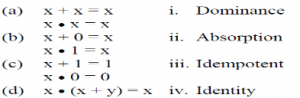A a-iii, b-iv, c-i, d-ii B a-iv, b-iii, c-i, d-ii C a-iv, b-iii, c-ii, d-i D a-iii, b-iv, c-ii, d-i
Digital-Logic-Design       Boolean-Algebra       UGC NET CS 2013 Sep-paper-2
Question 45 Explanation:
Idempotent laws: x+x=x and x.x=x
Identity laws: x+0=x and x.1=x
Dominance laws: x+1=1 and x.0=0
Absorption laws: x.(x+y)=x
 Question 46
The absorption law in Boolean algebra say that
 A X + X = X B X . X = X C x + x . y = x D None of the above
Digital-Logic-Design       Boolean-Algebra       UGC NET CS 2011 June-Paper-2
Question 46 Explanation:
Absorption laws in Boolean algebra:
X • (X+Y) = X
X + X•Y = X
 Question 47
The idempotent law in Boolean algebra says that :
 A ~(~x)=x B x+x=x C x+xy=x D x(x+y)=x
Digital-Logic-Design       Boolean-Algebra       UGC NET CS 2008-june-Paper-2
Question 47 Explanation:
Idempotent law:
The idempotence in the context of elements of algebras that remain invariant when raised to a positive integer power, and literally means "(the quality of having) the same power", from idem + potence (same + power).
(i). X + X=X
(ii). X ∧ X=X
According to boolean algebra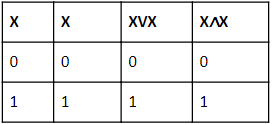Question 48
If p and q are two statements and they take truth values p = 1 and q = 1, then their conjunction p and q written as p ^ q takes truth value :
 A 0 B 1 C -1 D None
Digital-Logic-Design       Boolean-Algebra       TNPSC-2017-Polytechnic-CS
Question 48 Explanation:There are 48 questions to complete.